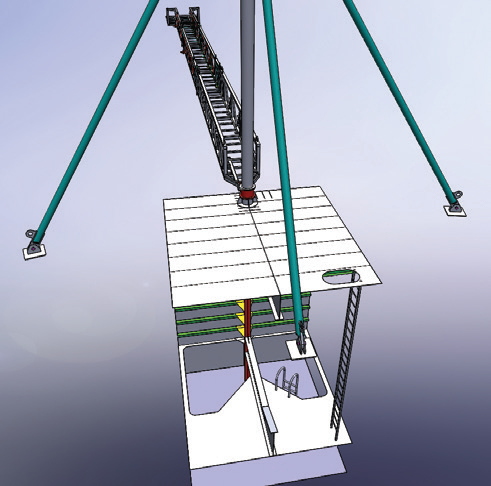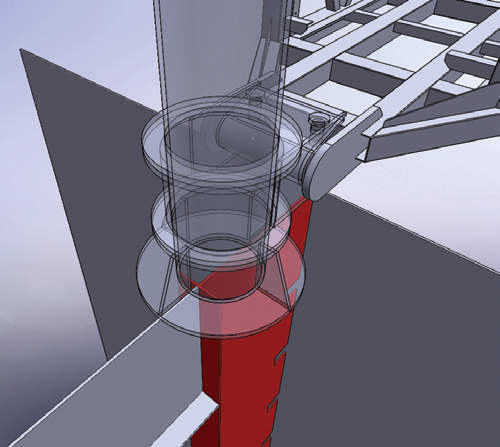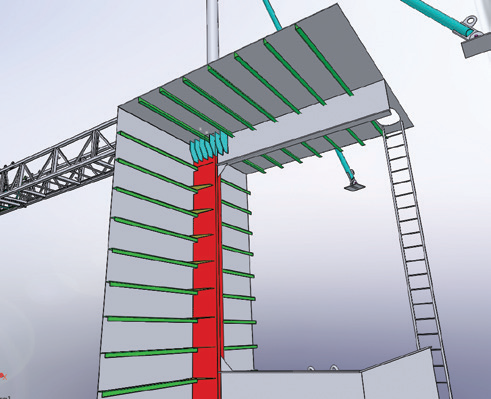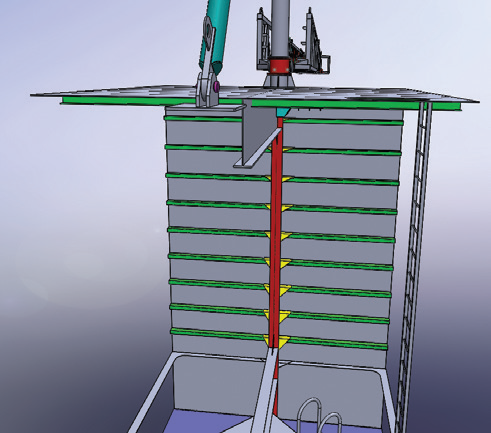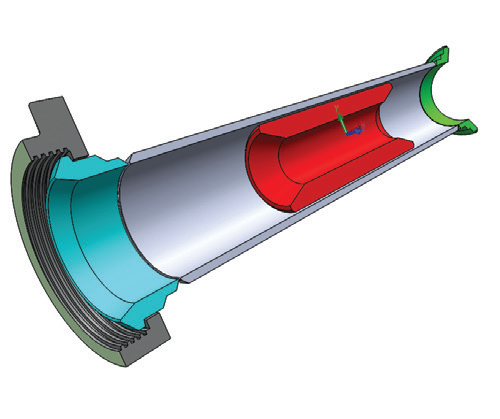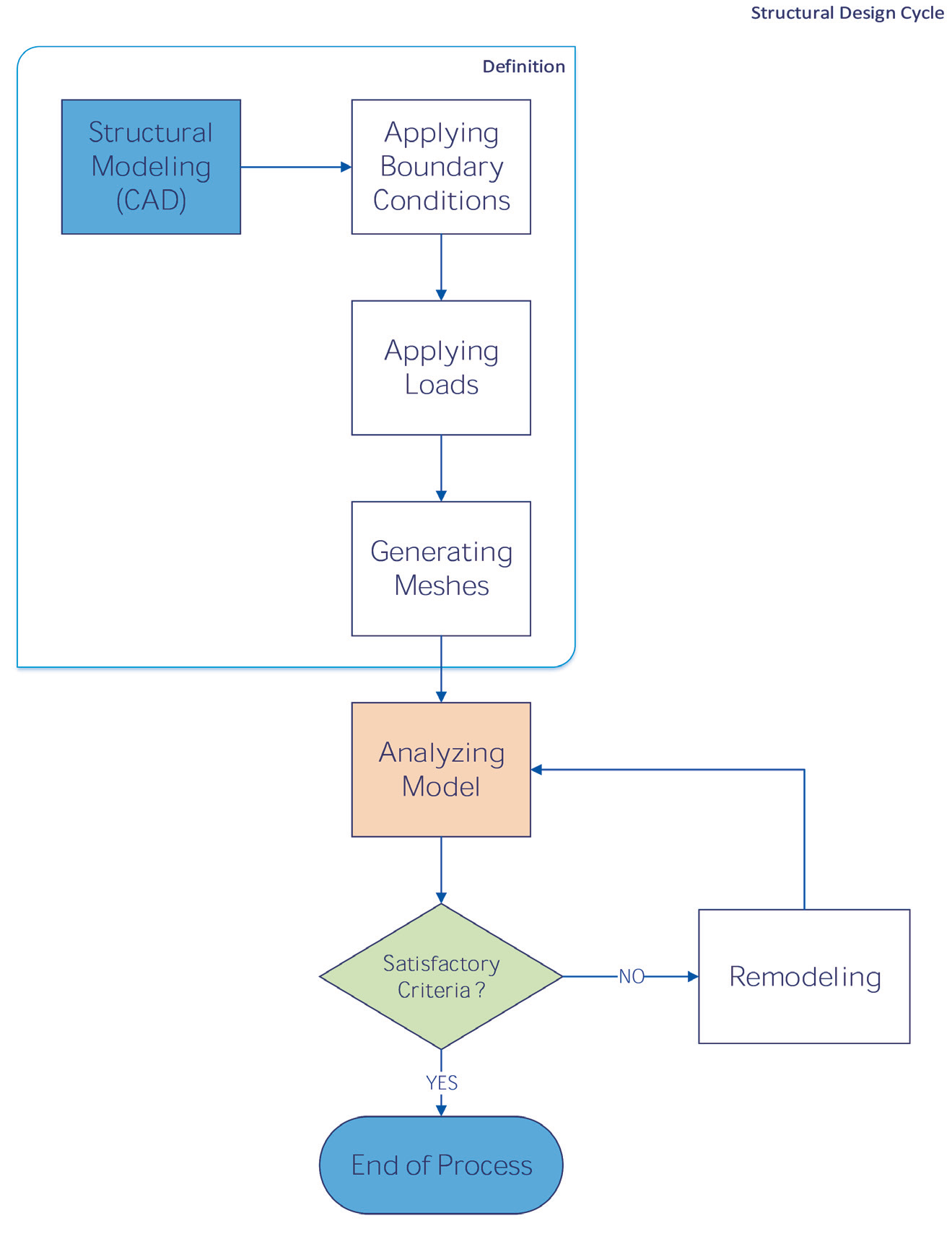Structural_Study

In order to design/modify a structure, regarding the loads and real life situation while utilizing, we carry out a structural study & analysis process to obtain the reaction forces, structural behavior while bearing loads and strength of the structure.

Structural analysis is the determination of the effects of loads on physical structures and their components. Structural analysis employs the fields of applied mechanics, materials science and applied mathematics to compute a structure’s deformations, internal forces, stresses, support reactions, accelerations, and stability. The results of the analysis are used to verify a structure’s fitness for use, often precluding physical tests. Structural analysis is thus a key part of the engineering design of structures.

One of the modern techniques used to simulate the structure’s behavior is FEM (Finite Element Method). The finite element method (FEM) is a numerical technique for finding approximate solutions to boundary value problems for partial differential equations. It is also referred to as finite element analysis (FEA). FEM subdivides a large problem into smaller, simpler parts, called finite elements. The simple equations that model these finite elements are then assembled into a larger system of equations that models the entire problem. FEM then uses variational methods from the calculus of variations to approximate a solution by minimizing an associated error function.

Nowadays, this method is vastly implemented in order to simulate many cases as follow:

• • Static Analysis
• • Thermal Analysis
• • Frequency Analysis
• • Buckling Analysis
• • Linear Dynamic
• • Drop/impact Test
• • Fatigue Analysis
• • Pressure Vessel Design
• • Nonlinear Analysis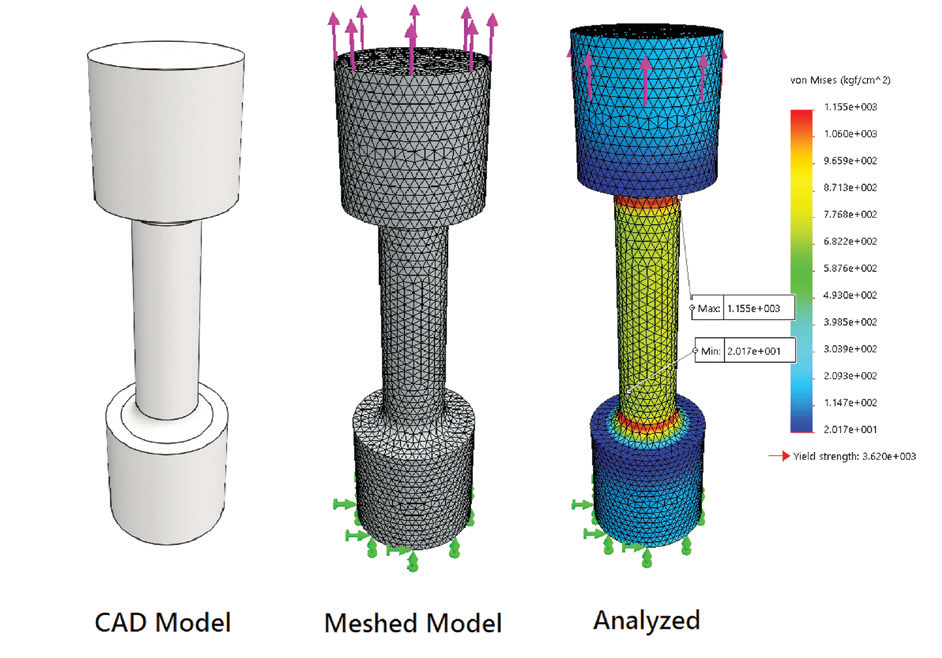We implement SolidWorks Premium 2016 & also SAP 2000 for Modeling, Analyzing and Optimizing the structures. To Prepare Shop-Drawings, SolidWorks or Tekla Structures (XSteel) will be used.